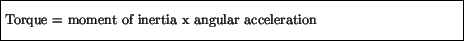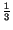Next: Rotational kinetic energy Up: Torque Previous: Static Equilibrium

## Moment of inertia

Newton's second law, Force = mass x acceleration, relates the acceleration that an object of a certain mass experiences when subject to a given force. There is an analogous relation between torque and angular acceleration, which introduces the concept of moment of inertia:Just as mass is a measure of how readily an object accelerates due to a given force, the moment of inertia of an object measures how easily an object rotates about a particular point of rotation. Thus, objects with a larger moment of inertia about a given point will be harder to rotate with a set torque. Correspondingly, a larger torque will cause a larger acceleration on a particular body.

The moment of inertia of a body, which is always measured relative to a point of rotation, depends in general on the object's mass and on its shape. It is perhaps evident that for a single mass going in a circle of fixed radius, the greater the radius the harder it is to change the angular velocity. This is because the actual displacement, and hence linear velocity of the mass is proportional to the radius, so greater radius, for a given angular displacement means greater linear displacement. In an extended object the parts that are further from the axis of rotation contribute more to the moment of inertia than the parts closer to the axis. So as a general rule, for two objects with the same total mass, the object with more of the mass located further from the axis will have a greater moment of inertia. For example, the moment of inertia of a solid cylinder of mass M and radius R about a line passing through its center isMR2, whereas a hollow cylinder with the same mass and radius has a moment of inertia of MR2. Similarly when a spinning figure skater pulls her arms in to her body she places more of her body weight closer to the axis of rotation and decreases her moment of inertia.Next: Rotational kinetic energy Up: Torque Previous: Static Equilibrium
modtech@theory.uwinnipeg.ca
1999-09-29